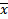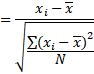Choose Language Hide Translation Bar
Highlighted

## How do I calculate z-scores at different aggregation levels?

Hello,

How do I use JSL to calculate Z-scores at different levels of aggregation? I am working with cancer mortality data (1999 - 2015) at the county-level. I need to calculate Z-scores to compare each county to the others within a state and all counties within the country. I cannot simply use the standardize function because it automatically takes an average of rates--which is invalid unless the populations are all exactly equal in size. I need to use some variant of the common Z-score formula to replace the mean of the rates for a given state (incorrect) with a mean of an aggregated region (entire state, for example). This is calculated by aggregating the numerator and denominator independently and then dividing:

in other words, SUM(All deaths in all counties in state K at year X) / SUM(All people in all counties in State K at  year X) =/= MEAN(Cancer Death Rates) for all counties in State X at time Y.

• Most critically, in the z-score calculation, the subtrahend in the numerator must reflect an aggregate mean for an entire region, NOT an average of Rates.
• The script needs to account for null values in its calculation of N and at different levels of aggregation (Number of counties for WV  for example = 55, number of counties for which data is non-null = 52; number of all counties in the US  = 3141, number of all counties for data is non-null = 2956, for example).
• the standardize function cannot be used here because theis calculated as an average of rates, which is invalid.Is there any way to use JSL to accomplish this task efficiently?

Article Labels

There are no labels assigned to this post.